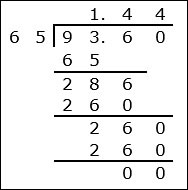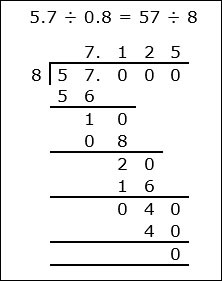# Decimal Division With Rounding

Here we consider division of decimals by decimals and then round the answer to nearest tenth, hundredth or as required.

Dividing and rounding to the nearest tenth

Here the long division is done so that the quotient has at least two places of decimals so that rounding can be done to the nearest tenth.

Dividing and rounding to the nearest hundredth

Here the long division is done so that the quotient has at least three places of decimals so that rounding can be done to the nearest hundredth.

Divide and round to the nearest tenth

9.36 ÷ 6.5

### Solution

Step 1:

Shifting decimal point one place to the right in both numbers

9.36 ÷ 6.5 = 93.6 ÷ 65Step 2:

Rounding the quotient to the nearest tenth, we get

9.36 ÷ 6.5 = 93.6 ÷ 65 = 1.44 ≈ 1.4

Divide and round to the nearest hundredth

5.7 ÷ 0.8

### Solution

Step 1:

Shifting decimal point one place to the right in both numbers

5.7 ÷ 0.8 = 57 ÷ 8

Step 2:Rounding the quotient to the nearest hundredth, we get

5.7 ÷ 0.8 = 57 ÷ 8 = 7.125 ≈ 7.13